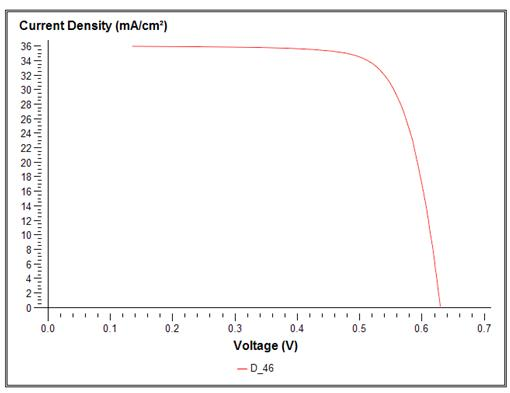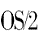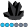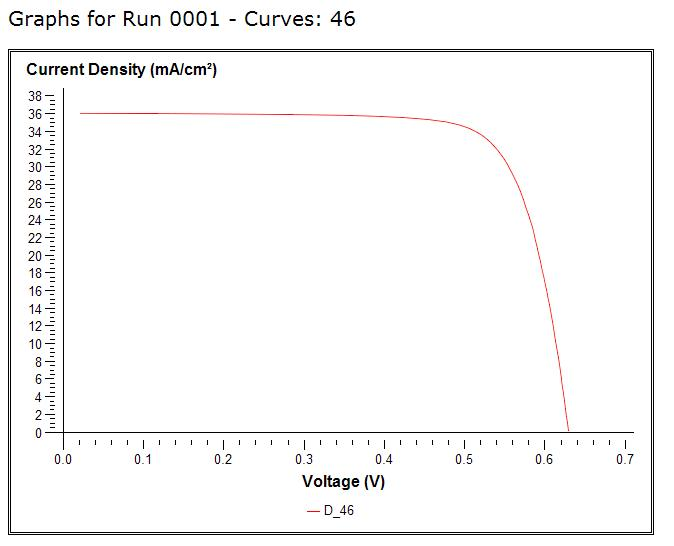## proc gplot does not draw all paointsMy axis goes fromzero to the maximum of V.

The problem is this does not prints the points from 0.0 to0.135 for V

/* Define symbolcharacteristics */

axis1label=("Current Density(mA/cm²)")

width=2

style=0

order=(0to &DmaxValue by 2);

axis2

label=("Voltage(V)")

width=2

style=0

order=(0to &VmaxValue by 0.1);

procgplot

data=all_data;

plot&plotstatement   /overlaylegend=legend1

href=0 vref=0 vaxis=axis1 haxis=axis2;

;

run;

4 REPLIES 4OS2Rules
Obsidian | Level 7

## Re: proc gplot does not draw all paoints

Hi:

Firstly, i would suggest that you increase the value of &DmaxValue for the Y axis by 1 (or 2) because it looks like the axis only goes to 36 and the data values are greater than that.  This would cause the line on the graph to start at the first X value that is < 36.

If that doesn't work, then include sample data and the rest of the code so we can see what is going on.

## Re: proc gplot does not draw all paoints

I changed my code a little bit.

proc sql; select max(Vmax)+0.1  into:VmaxValue from all_data_vmax; quit;

%put &VmaxValue;

It was already +1 in fact..

proc sql; select max(Dmax)+3  into:DmaxValue from all_data_vmax; quit;

%put &DmaxValue;

/* Define symbol characteristics */

axis1 label=("Current Density (mA/cm²)")

width=2

style=0

order=(0 to &DmaxValue by 2);

axis2

label=("Voltage (V)")

width=2

style=0

order=(0 to &VmaxValue by 0.1);

proc gplot

data=all_data;

plot &plotstatement   /overlay legend=legend1

href=0 vref=0 vaxis=axis1 haxis=axis2;

;

run;

And result: Better: But not yet perfect...OS2Rules
Obsidian | Level 7

## Re: proc gplot does not draw all paoints

Without seeing all the data, I can only guess: it looks like there are values of X that are less than 0 (zero).  I think you need to adjust the range of the axis to begin with the lowset value of X.  You may need another macro variable for the min(x) value.

If you want the line to start with X=0, then you will need to insure that there is a data point with X=0, otherwise the line will start with the lowest value of X > 0 (which looks like obs 98 where x=0.02099 something - which is where the line seems to start on your graph).

## Re: proc gplot does not draw all paoints

It's difficult to tell without having all the data, to experiment with the code, but I'm supposing you are running into a couple of problems/features...

When you're specifying that the axis should go 'by 2', then if your maximum Y-value is not a mulitple of 2, then your axis is going to get cut short.  Here is a small example that demonstrates this (note that although I specify 'to 5', the axis only goes to 4, since that is the maximum multiple of 2 that is within the specified range:

data foo;
x=1; y=1; output;
run;

axis1 order=(0 to 5 by 2);

proc gplot data=foo;
plot y*x / vaxis=axis1;
run;

Also, when you're using an interpolation to connect the points, it only connects the points that fall within the specified/visible axis range.  If you want points outside the visible axis range to be considered in the interpolation, you can use the mode=include on the symbol statement:

http://support.sas.com/documentation/cdl/en/graphref/63022/HTML/default/viewer.htm#symbolchap.htm

My recommendation? - Let the axes auto-scale, and they will always pick "reasonably good" min/max & tickmarks, and all the data points will always be in the graphDiscussion stats
• 4 replies
• 787 views
• 0 likes
• 3 in conversation